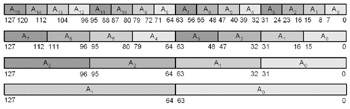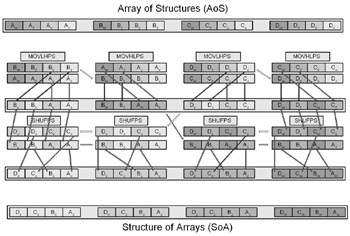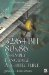# Chapter 6: Data Conversion## Data Interlacing, Exchanging, Unpacking, and Merging

Data must sometimes be interlaced to get it into a form that can be easily handled. By understanding how to interlace and de-interlace data, a most productive solution can be found for solving an expression.

The instructions in this chapter are easier to understand through visualization, and each processor has its set of instructions that it handles, but here is where data swizzling can easily be confusing: converting data from the output of one instruction and used as the input of another.Figure 6-1: Little-endian data conversion

As a guide to assist you in remembering big- versus little-endian orientations, the following are the placement of bytes within the 64-bit data diagrams used in this chapter. Keep in mind that each 64-bit block is a repeat of the previous block.

Little-Endian

` 0x88,0x99,0xaa,0xbb,0xcc,0xdd,0xee,0xff   (8-bit)   0x9988,   0xbbaa,   0xddcc,   0xffee    (16-bit)      0xaab9988,          0xffeeddcc       (32-bit) `

Big-Endian

` 0x88,0x99,0xaa,0xbb,0xcc,0xdd,0xee,0xff   (8-bit)   0x8899,   0xaabb,   0xccdd,   0xeeff    (16-bit)      0x8899aabb,          0xccddeeff      (32-bit) `

The one thing to remember here is that the data elements are isolated from each other. The A n placement of each element is related to its position. For example, when related to a quad vector:

A : A x , A 1 : A y , A 2 : A z , and A 3 : A w .

So that means that A w A z A y A x are visually on the far right just like A 3 A 2 A 1 A for little-endian, and A x ,A y ,A z ,A w are on the far left just like A , A 1 , A 2 , A 3 for big-endian.

As long as you get the element positions correct for your processor, then the data flow represented by the arrows in the diagrams will be correct.

 Note The bit indicators on the diagrams in this section are in little-endian byte order.

Quite often, data needs to be migrated from one form to another, and a single instruction may not be sufficient. For instance, a matrix is made up of four vectors: A xyzw ,B xyzw , C xyzw , D xyzw . This is known as an Array of Structures (AoS). But mathematical operations are typically between like terms such as A x B x C x D x ,A y B y C y D y , etc. This is known as a Structure of Arrays (SoA), which is more matrix friendly (and efficient) due to the simultaneous operation upon the same elements. To get the data from one form to another requires the data to be manipulated.

The following is one such example.Figure 6-2: AoS to SoA SIMD conversion32/64-Bit 80x86 Assembly Language Architecture
ISBN: 1598220020
EAN: 2147483647
Year: 2003
Pages: 191

Similar book on Amazon